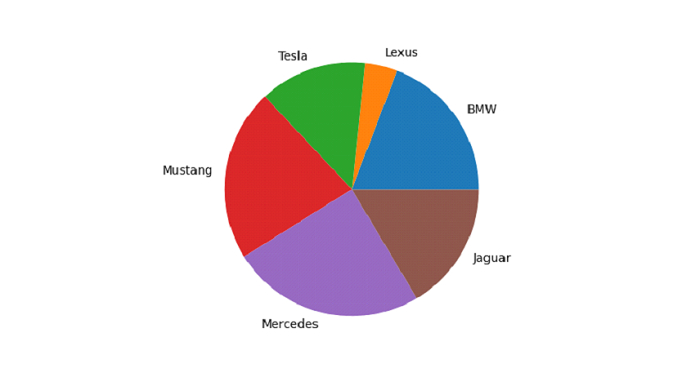# Python - Plot a Pie Chart for Pandas Dataframe with Matplotlib?

To plot a Pie Chart, use the plot.pie(). The pie plot is a proportional representation of the numerical data in a column.

Import the required libraries −

import pandas as pd
import matplotlib.pyplot as plt

Create a DataFrame −

dataFrame = pd.DataFrame({
"Car": ['BMW', 'Lexus', 'Tesla', 'Mustang', 'Mercedes', 'Jaguar'],"Reg_Price": [7000, 1500, 5000, 8000, 9000, 6000]
})

Plot a Pie Chart for Registration Price column with label Car column −

plt.pie(dataFrame["Reg_Price"], labels = dataFrame["Car"])


## Example

Following is the code −

import pandas as pd
import matplotlib.pyplot as plt

# creating dataframe
dataFrame = pd.DataFrame({
"Car": ['BMW', 'Lexus', 'Tesla', 'Mustang', 'Mercedes', 'Jaguar'],"Reg_Price": [7000, 1500, 5000, 8000, 9000, 6000]
})

# plot a Pie Chart for Registration Price column with label Car column
plt.pie(dataFrame["Reg_Price"], labels = dataFrame["Car"])
plt.show()

## Output

This will produce the following output −